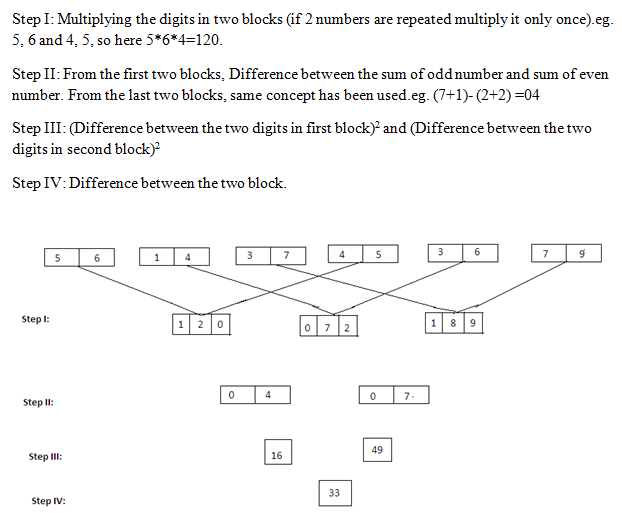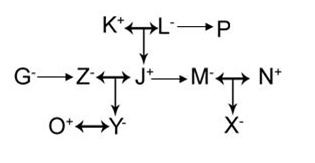# RRB PO Mains Reasoning (Day-01)

## Reasoning Materials for RRB PO Mains:

Dear Aspirants, Our IBPS Guide team is providing new series of Reasoning Questions for RRB Po Mains 2020 so the aspirants can practice it on a daily basis. These questions are framed by our skilled experts after understanding your needs thoroughly. Aspirants can practice these new series questions daily to familiarize with the exact exam pattern and make your preparation effective.

Start Quiz

Input output

Direction (1-5): Study the following information carefully and answer the questions given below:

An input-output is given in different steps. Some mathematical operations are done in each step. No mathematical operation is repeated in next step but it can be repeated with some other mathematical operation (as multiplication can be used with addition in step I and same can be used with subtraction in step II)

1) Which of the following is the sum of two blocks in step II?

A) 18

B) 16

C) 15

D) 11

E) None of these

2) which of the following is the resultant after dividing the first block (consider 3 digits as a whole number) in step I from the left end by the second digit of the first block from the left end in step II?

A) 20

B) 30

C) 3

D) 10

E) None of these

3) What will be the remainder if we divide the second block from the left end with the first block from the left end in step III?

A) 3

B) 1

C) 2

D) 0

E) None of these

4) What is the difference between the third digit of the second block in step I and second digit of the second block from the left end in step II?

A) 3

B) 9

C) 5

D) 4

E) None of these

5) What is the sum of the digits of third block from the left end in step I?

A) 18

B) 16

C) 17

D) 15

E) None of these

Coded blood relation

Direction (6-9): Study the following information and answer the questions given below:

‘A#B’ means ‘A and B are siblings’

[email protected]’ means ‘A and B are married couples’

‘A*B’ means ‘B is the only daughter of A’

‘A%B’ means ‘B is the father of A’

‘A^B’ means ‘B is the sister in law of A’

‘A-B’ means ‘B is the brother in law of A’

II) J#M*X

V) P#L*M

VI) X%N-J

VII) G#Z*Y

6) How is K related to N, according to the above expression?

A) Father-in-law

B) Mother-in-law

C) Brother-in-law

D) Sister-in-law

E) None of these

7) Which of the following is definitely true according to the above expression?

A) J and X are males

B) P has two children

C) X is the nephew of J

D) Y is the niece of M

E) None of these

8) How many female members are there in the family?

A) Four

B) Five

C) Six

D) Cannot be determined

E) None of these

9) How is J related to P according to the above expression?

A) Niece

B) Nephew

C) Aunt

D) Uncle

E) None of these

Critical reasoning

Directions (10): The following questions consist of a statement followed by two arguments I and II. Find which of the following statement(s) is/are strong.

Statements: According to a survey in most of the rivers, the amount of oxygen in a litre of water has decreased to 0.1 cubic centimetres only, while this average in 1940 was around 2.5 cubic centimetres.

Arguments:

I) Fish and other aquatic organisms start dying due to lack of oxygen in the polluted water.

II) Hydrocarbons in the oil spread on the surface of the oceans due to which marine and aquatic organisms do not get the oxygen and they die consequently.

A) Only argument I is strong

B) Only argument II is strong

C) Either argument I or II is strong

D) Neither argument I nor II is strong

E) Both argument I and II are strong

Directions (1-5):Directions (6-9):